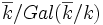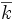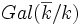# Max-spectrum of polynomial ring over a field

You might be looking for: max-spectrum of multivariate polynomial ring over a field

Let$k$ be a field. The max-spectrum of the polynomial ring$k[x]$ can be described as follows.

## As a set

Here are some descriptions:

• It is the set of all irreducible monic polynomials in$k[x]$. A particularly distinguished class of irreducible polynomials is the monic linear polynomials, which can be identified with the points of$k$ itself. When$k$ is algebraically closed, these are the only elements.
• It is the set$\overline{k}/Gal(\overline{k}/k)$. Here$\overline{k}$ denotes the algebraic closure of$k$ and$Gal(\overline{k}/k)$ is the group of automorphisms. The notation here means that we take points of$\overline{k}$, and identify those which have the same minimal polynomial. Note that distinct elements of$k$ remain distinct, and when$k$ itself is algebraically closed, these are the only elements.

Note that the max-spectrum of the polynomial ring over any field is always infinite, whether or not the field is algebraically closed and whether or not the field is finite. This is because there are always infinitely many irreducible polynomials.

## As a topological space

The max-spec topology is the cofinite topology: the proper closed subsets are precisely the finite ones. Thus, the space is a T1 space and is also irreducible and Noetherian.